View Rules | View Capture Matches

The scoring system may seem a bit complex at first, so be sure to check the scoring breakdown for the cards of you and your opponent. You can do so at any point in the game. Click on a player's score to see their scoring breakdown.

Cards that belong to the same group for matching and capturing do not necessarily belong in the same group for scoring. Points are scored by capturing the following combinations of cards:

NEOPETS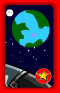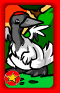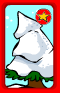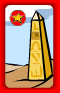Set of 5 = 15 points
Set of 4 = 4 points
Set of 3, not including the Haunted Woods Neopets Card = 3 points
Set of 3, including the Haunted Woods card = 2 points

PETPET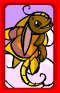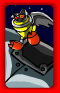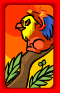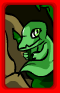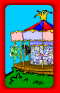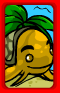Set of 5 = 1 point
Each additional over 5 = 1 point

Flying PetpetSet of 3 = 5 points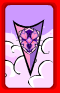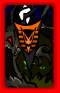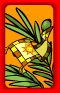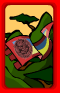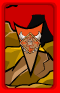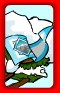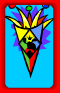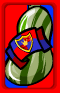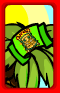Set of 5 = 1 point
Each additional beyond 5 = 1 pointSet of 3 = 3 pointsSet of 3 = 3 pointsSet of 3 = 3 points

NEOPIAN WORLD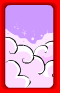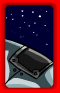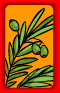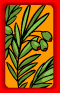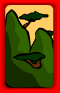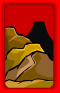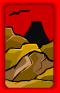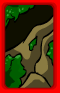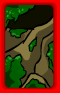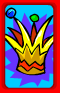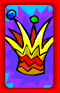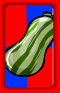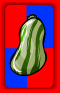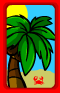Set of 10 = 1 point
Each additional beyond 10 = 1 point

The following Neopian World cards count as two cards:This card can be used as either a Petpet or Neopian World card for scoring: